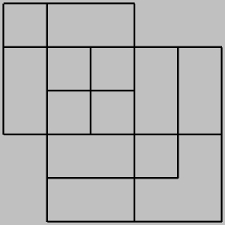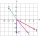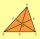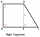# Coordinates of square vertices

The ABCD square has the center S [−3, −2] and the vertex A [1, −3]. Find the coordinates of the other vertices of the square.

x1 =  -7
y1 =  -1
x2 =  -2
y2 =  2
x3 =  -4
y3 =  -6

### Step-by-step explanation:

${y}_{1}={s}_{1}-{d}_{1}=\left(-2\right)-\left(-1\right)=-1$
${x}_{2}={s}_{0}+{n}_{0}=\left(-3\right)+1=-2$
${y}_{2}={s}_{1}+{n}_{1}=\left(-2\right)+4=2$
${x}_{3}={s}_{0}-{n}_{0}=\left(-3\right)-1=-4$
${y}_{3}={s}_{1}-{n}_{1}=\left(-2\right)-4=-6$Did you find an error or inaccuracy? Feel free to write us. Thank you!Tips to related online calculators
Line slope calculator is helpful for basic calculations in analytic geometry. The coordinates of two points in the plane calculate slope, normal and parametric line equation(s), slope, directional angle, direction vector, the length of the segment, intersections of the coordinate axes, etc.
Our vector sum calculator can add two vectors given by their magnitudes and by included angle.

#### You need to know the following knowledge to solve this word math problem:

We encourage you to watch this tutorial video on this math problem:

## Related math problems and questions:

• Vector perpendicularFind the vector a = (2, y, z) so that a⊥ b and a ⊥ c where b = (-1, 4, 2) and c = (3, -3, -1)
• Coordinates of square verticesI have coordinates of square vertices A / -3; 1/and B/1; 4 /. Find coordinates of vertices C and D, C 'and D'. Thanks Peter.
• TrianglePlane coordinates of vertices: K[11, -10] L[10, 12] M[1, 3] give Triangle KLM. Calculate its area and its interior angles.
• VectorsVector a has coordinates (8; 10) and vector b has coordinates (0; 17). If the vector c = b - a, what is the magnitude of the vector c?
• Space vectors 3DThe vectors u = (1; 3; -4), v = (0; 1; 1) are given. Find the size of these vectors, calculate the angle of the vectors, the distance between the vectors.
• 3d vector componentThe vector u = (3.9, u3) and the length of the vector u is 12. What is is u3?
• SquarePoints A[9,9] and B[-4,1] are adjacent vertices of the square ABCD. Calculate the area of the square ABCD.
• Angle of the body diagonalsUsing vector dot product calculate the angle of the body diagonals of the cube.
• Find the 5Find the equation of the circle with center at (1,20), which touches the line 8x+5y-19=0
• Scalar productCalculate the scalar product of two vectors: (2.5) (-1, -4)
• CenterIn the triangle ABC is point D[1,-2,6], which is the center of the |BC| and point G[8,1,-3], which is the center of gravity of the triangle. Find the coordinates of the vertex A[x,y,z].
• Parametric formCalculate the distance of point A [2,1] from the line p: X = -1 + 3 t Y = 5-4 t Line p has a parametric form of the line equation. ..
• Coordinates of a centroindLet’s A = [3, 2, 0], B = [1, -2, 4] and C = [1, 1, 1] be 3 points in space. Calculate the coordinates of the centroid of △ABC (the intersection of the medians).
• Unit vector 2DDetermine coordinates of unit vector to vector AB if A[-6; 8], B[-18; 10].
• Calculate 7Calculate the height of the trapezoid ABCD, where coordinates of vertices are: A[2, 1], B[8, 5], C[5, 5] and D[2, 3]
• Perpendicular projectionDetermine the distance of a point B[1, -3] from the perpendicular projection of a point A[3, -2] on a straight line 2 x + y + 1 = 0.
• Vector v4Find the vector v4 perpendicular to vectors v1 = (1, 1, 1, -1), v2 = (1, 1, -1, 1) and v3 = (0, 0, 1, 1)